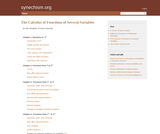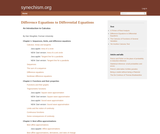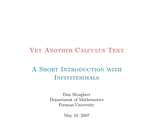# 4 Results

View
Selected filters:
• Furman UniversityConditional Remix & Share Permitted
CC BY-NC-SA
Rating

This books covers the following topics: Geometry of Rn; functions from R to Rn; functions from Rn to R; functions from Rm to Rn.

Subject:
Calculus
Material Type:
Homework/Assignment
Textbook
Provider:
Furman University
Author:
Dan Sloughter
04/25/2019Conditional Remix & Share Permitted
CC BY-NC-SA
Rating

This book covers the following topics: Sequences, limits, and difference equations; functions and their properties; best affine approximations; integration; polynomial approximations and Taylor series; transcendental functions; the complex plane; differential equations.

Subject:
Mathematics
Material Type:
Textbook
Provider:
Furman University
Author:
Sloughter, Dan
04/25/2019Conditional Remix & Share Permitted
CC BY-NC-SA
Rating

A discussion of how small probabilities license statistical inferences, and how frequentists C. S. Peirce, R. A. Fisher, J. Neyman, E. S. Pearson, and D. Mayo differ in their interpretations of the p-value of a statistical test. (Unpublished paper)

Subject:
Statistics and Probability
Material Type:
Provider:
Furman University
Author:
Sloughter, Dan
04/25/2019Conditional Remix & Share Permitted
CC BY-NC-SA
Rating

I intend this book to be, firstly, a introduction to calculus based on the hyperreal number system. In other words, I will use infinitesimal and infinite numbers freely. Just as most beginning calculus books provide no logical justification for the real number system, I will provide none for the hyperreals. The reader interested in questions of foundations should consult books such as Abraham Robinson's Non-standard Analysis or Robert Goldblatt's Lectures on the Hyperreals. Secondly, I have aimed the text primarily at readers who already have some familiarity with calculus. Although the book does not explicitly assume any prerequisites beyond basic algebra and trigonometry, in practice the pace is too fast for most of those without some acquaintance with the basic notions of calculus.

1 Derivatives

1.2 Rates of change
1.3 The hyperreals
1.4 Continuous functions
1.5 Properties of continuous functions
1.6 The derivative
1.7 Properties of derivatives
1.8 A geometric interpretation of the derivative
1.9 Increasing, decreasing, and local extrema
1.10 Optimization
1.11 Implicit differentiation and rates of change
1.12 Higher-order derivatives
2 Integrals

2.1 Integrals
2.2 Definite integrals
2.3 Properties of definite integrals
2.4 The fundamental theorem of integrals
2.5 Applications of definite integrals
2.6 Some techniques for evaluating integrals
2.7 The exponential and logarithm functions
Index

Access also available here: http://www.synechism.org/wp/yet-another-calculus-text/

Subject:
Calculus
Material Type:
Textbook
Provider:
Furman University
Author:
Dan Sloughter